﻿ Question on Ultrasonic Ranging Module HC-SR04 - Beta Firmware and Drivers - Netduino Forums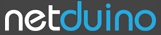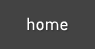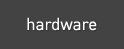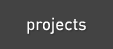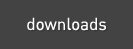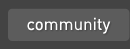The Netduino forums have been replaced by new forums at community.wildernesslabs.co. This site has been preserved for archival purposes only and the ability to make new accounts or posts has been turned off.# Question on Ultrasonic Ranging Module HC-SR04

driver sonar

Best Answer baxter, 04 March 2013 - 09:17 PM

I am using code from a different reference, but it works well for me. The interrupt is triggered on the rising edge of the echo return pulse and the ticks are saved. On the falling edge, the elapsed ticks are computed and the difference is the pulse width ( See sub Trigger to generate the trigger pulse).

I translated this from C# to VB, but the link in the code is in C#.

`Imports System.ThreadingImports Microsoft.SPOTImports Microsoft.SPOT.HardwareImports SecretLabs.NETMF.HardwareImports SecretLabs.NETMF.Hardware.NetduinoImports System.Math''http://forums.netduino.com/index.php?/topic/2202-measures-jumping/' Written by BG on July the 25th 2011 to test the HC-SR04 sensor (above Ref.)' Physical Placement 240 mm perpendicular to flat reflective surface.' Physical distance is measured from 1/2 the height of cylindrical sensor case to flat surface.'100 measurements from Netduino Plus 2'====================================='Calculated Distance = 240.79349999999999 mm'Mean = 241.02483673469382  Standard Deviation = 1.0885082552654264'Low pass filter distance (alpha=0.3) = 240.78816817382358'Max/Min on interval: MAX = 245.08 mm, MIN = 240.62 mm'100 measurementsModule Ultrasonic_Dist_Sensor    Dim ConvFactor As Double 'conversion factor: Echo pulse width ticks to distance    Dim Distance As Double = 0    Dim ticks As Long    Private EchoPin As New InterruptPort(Pins.GPIO_PIN_D10, True, Port.ResistorMode.Disabled, Port.InterruptMode.InterruptEdgeBoth)    Private TriggerPin As New OutputPort(Pins.GPIO_PIN_D12, False)    Public Sub Main()        'compute the mean and standard deviation of the measurements        Dim MeanVariance As Smooth = New Smooth(0) ' initialize time series        Dim ExpSmooth As Double        'conversion factor for return pulse ticks to mm.         ConvFactor = ConversionFactor(20.0) 'call with temperature (deg C)        'handler to process return pulse        AddHandler EchoPin.OnInterrupt, AddressOf Echo_OnInterrupt 'echo        EchoPin.DisableInterrupt()        Dim count As Integer = 0        While True            Trigger() 'trigger pulse            Thread.Sleep(1000)            Debug.Print("Distance = " & Distance.ToString & "  mm")            MeanVariance.MeanVarAddData(Distance)            ExpSmooth = MeanVariance.LowPassFilter(Distance, 0.3)            If (count > 3) Then ' first collect some data before printing                Debug.Print("Mean = " & MeanVariance.mean.ToString &                             "  Standard Deviation = " & MeanVariance.StdDeveviation.ToString)                Debug.Print("Low pass filter distance (alpha=0.3) = " &                           ExpSmooth.ToString)            End If            count += 1            If (count = 103) Then Exit While        End While    End Sub    Public Function ConversionFactor(Temp As Double) As Double        'Temp = input degrees Celsius        'The approximate speed of sound in dry air is given by the formula:        'c = 331.5 + 0.6 * [air temperature in degrees Celsius]        'At 20�C, c = 331.5 + 0.6 * 20 = 343.5 m/s        '1 tick = 0.1uSec, 1 meter = 1000 mm, Hence        'c = c * 1000 / 10000000 --> c mm/tick        'round trip out and back so divide by 2 to get distance        Dim c As Double = 331.5 + 0.6 * Temp        Return (c * 1000 / 10000000) / 2.0    End Function    Public Sub Trigger()        EchoPin.EnableInterrupt()        TriggerPin.Write(False)        Thread.Sleep(2)        TriggerPin.Write(True)        Thread.Sleep(15)        TriggerPin.Write(False)        Thread.Sleep(4)    End Sub    Sub Echo_OnInterrupt(port As UInteger, state As UInteger, time As DateTime)        If state = 0 Then            ' falling edge, end of pulse            Dim pulseWidth As Integer = CInt(time.Ticks - ticks)            Distance = ConvFactor * pulseWidth        Else            ticks = CInt(time.Ticks) 'rising edge        End If        EchoPin.ClearInterrupt()        Thread.Sleep(50)    End SubEnd Module`

Go to the full post1 reply to this topic

### #1martinlee556

martinlee556

Posted 02 March 2013 - 05:59 PM

Hi all,

I would like to write my own class/driver for this module HC-SR04, datasheet link as below.

http://www.micropik.com/PDF/HCSR04.pdf

Although I know there is someone already posted some working code(link below) for this module, but I still very curious about on how to write it.

In the datasheet, it says "You only need to supply a short 10uS pulse to the trigger input to start the ranging".

Now, my question is how to translate this sentence into C# code?

It looks to me that I will need to use PWM object to send a message to this module so it can be triggered.

`PWM pwm = new PWM(PWMChannels.PWM_PIN_D5, ??, ??, PWM.ScaleFactor.Microseconds, false);`

The missing parameters above are Period and Duration.

How do I set it so it will gernerate a 10us pulse to trigger the module??  Am I on the right track??

I am a newbie on electronics. Would appreciate if you can explain in the way I can understand.

By the way, I am using Netduino Plus 2 with .Net MF v4.2.

Thank you in advanced.

### #2baxter

baxter

Posted 04 March 2013 - 09:17 PM   Best Answer

I am using code from a different reference, but it works well for me. The interrupt is triggered on the rising edge of the echo return pulse and the ticks are saved. On the falling edge, the elapsed ticks are computed and the difference is the pulse width ( See sub Trigger to generate the trigger pulse).

I translated this from C# to VB, but the link in the code is in C#.

`Imports System.ThreadingImports Microsoft.SPOTImports Microsoft.SPOT.HardwareImports SecretLabs.NETMF.HardwareImports SecretLabs.NETMF.Hardware.NetduinoImports System.Math''http://forums.netduino.com/index.php?/topic/2202-measures-jumping/' Written by BG on July the 25th 2011 to test the HC-SR04 sensor (above Ref.)' Physical Placement 240 mm perpendicular to flat reflective surface.' Physical distance is measured from 1/2 the height of cylindrical sensor case to flat surface.'100 measurements from Netduino Plus 2'====================================='Calculated Distance = 240.79349999999999 mm'Mean = 241.02483673469382  Standard Deviation = 1.0885082552654264'Low pass filter distance (alpha=0.3) = 240.78816817382358'Max/Min on interval: MAX = 245.08 mm, MIN = 240.62 mm'100 measurementsModule Ultrasonic_Dist_Sensor    Dim ConvFactor As Double 'conversion factor: Echo pulse width ticks to distance    Dim Distance As Double = 0    Dim ticks As Long    Private EchoPin As New InterruptPort(Pins.GPIO_PIN_D10, True, Port.ResistorMode.Disabled, Port.InterruptMode.InterruptEdgeBoth)    Private TriggerPin As New OutputPort(Pins.GPIO_PIN_D12, False)    Public Sub Main()        'compute the mean and standard deviation of the measurements        Dim MeanVariance As Smooth = New Smooth(0) ' initialize time series        Dim ExpSmooth As Double        'conversion factor for return pulse ticks to mm.         ConvFactor = ConversionFactor(20.0) 'call with temperature (deg C)        'handler to process return pulse        AddHandler EchoPin.OnInterrupt, AddressOf Echo_OnInterrupt 'echo        EchoPin.DisableInterrupt()        Dim count As Integer = 0        While True            Trigger() 'trigger pulse            Thread.Sleep(1000)            Debug.Print("Distance = " & Distance.ToString & "  mm")            MeanVariance.MeanVarAddData(Distance)            ExpSmooth = MeanVariance.LowPassFilter(Distance, 0.3)            If (count > 3) Then ' first collect some data before printing                Debug.Print("Mean = " & MeanVariance.mean.ToString &                             "  Standard Deviation = " & MeanVariance.StdDeveviation.ToString)                Debug.Print("Low pass filter distance (alpha=0.3) = " &                           ExpSmooth.ToString)            End If            count += 1            If (count = 103) Then Exit While        End While    End Sub    Public Function ConversionFactor(Temp As Double) As Double        'Temp = input degrees Celsius        'The approximate speed of sound in dry air is given by the formula:        'c = 331.5 + 0.6 * [air temperature in degrees Celsius]        'At 20�C, c = 331.5 + 0.6 * 20 = 343.5 m/s        '1 tick = 0.1uSec, 1 meter = 1000 mm, Hence        'c = c * 1000 / 10000000 --> c mm/tick        'round trip out and back so divide by 2 to get distance        Dim c As Double = 331.5 + 0.6 * Temp        Return (c * 1000 / 10000000) / 2.0    End Function    Public Sub Trigger()        EchoPin.EnableInterrupt()        TriggerPin.Write(False)        Thread.Sleep(2)        TriggerPin.Write(True)        Thread.Sleep(15)        TriggerPin.Write(False)        Thread.Sleep(4)    End Sub    Sub Echo_OnInterrupt(port As UInteger, state As UInteger, time As DateTime)        If state = 0 Then            ' falling edge, end of pulse            Dim pulseWidth As Integer = CInt(time.Ticks - ticks)            Distance = ConvFactor * pulseWidth        Else            ticks = CInt(time.Ticks) 'rising edge        End If        EchoPin.ClearInterrupt()        Thread.Sleep(50)    End SubEnd Module`

### Also tagged with one or more of these keywords: driver, sonar

#### 0 user(s) are reading this topic

0 members, 0 guests, 0 anonymous usershome    hardware    projects    downloads    community    where to buy    contact Copyright © 2016 Wilderness Labs Inc.  |  Legal   |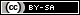This webpage is licensed under a Creative Commons Attribution-ShareAlike License.